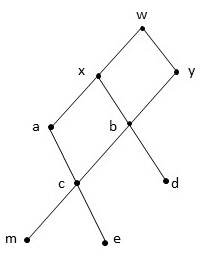# Given the following Hasse diagram, find a) minimal elements b) minimum, c) maximum

## Question:

Given the following Hasse diagram, find

a) minimal elements

b) minimum,

c) maximum## Hesse Diagrams

Hesse Diagrams represent partial order relations. The vertical position of elements has a meaning. Direction going upwards represents "greater than". Minimal elements are the ones on the bottom of the diagram. Maximal elements are the ones on the top. Maximum and Minimum are unique if they exist.

## Answer and Explanation:

(a) In this diagram we can see that the minimal elements, the ones on the bottom are "m" and "e".

(b) There is no minimum element since there are 2 minimal elements.

(c) The maximum is the unique maximal element, which is "w".

#### Learn more about this topic:Graph Theory Concepts and Terminology

from Math 106: Contemporary Math

Chapter 9 / Lesson 2
3.5K